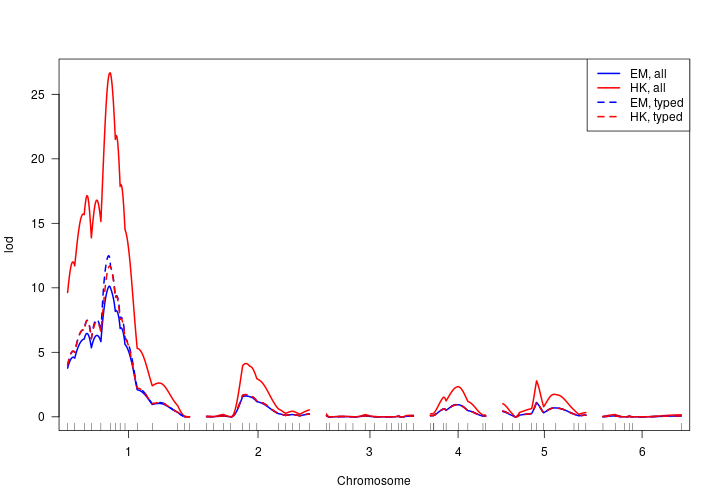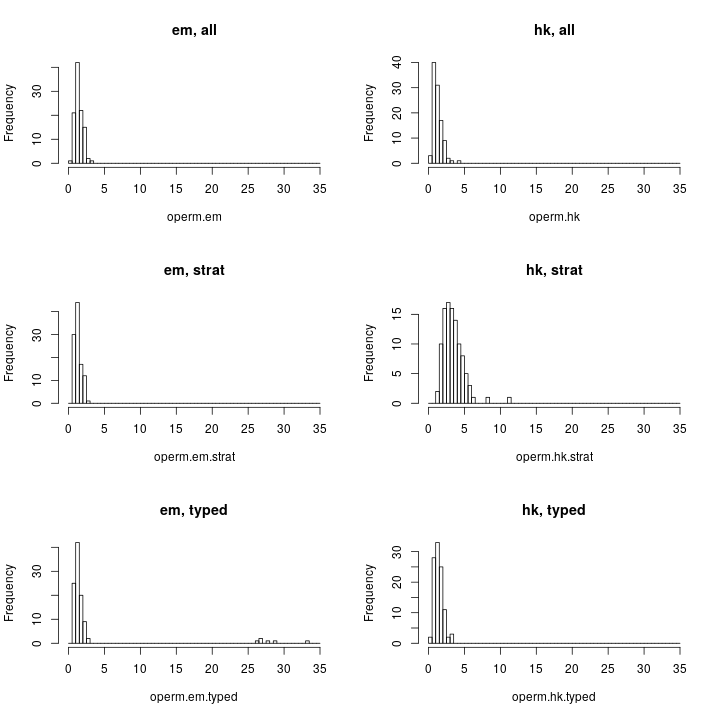### Homework #3: QTL mapping, solutions

#### Analyses

I'll do all of the calculations first, and then summarize them, and then discuss them.

I load the R/qtl package and the data, as follows:

``````library(qtl)
"hw3.csv")
``````

I'll first perform standard interval mapping (IM) and Haley-Knott regression.

``````hw <- calc.genoprob(hw, step=1)
out.em <- scanone(hw, method="em")
out.hk <- scanone(hw, method="hk")
``````

I then perform a standard permutation test by each method.

``````num_cores <- parallel::detectCores()
n.perm <- 100
operm.em <- scanone(hw, method="em", n.perm=n.perm, n.cluster=num_cores)
operm.hk <- scanone(hw, method="hk", n.perm=n.perm, n.cluster=num_cores)
``````

I then perform stratified permutation tests.

``````nt <- ntyped(hw)
strat <- as.numeric(nt > mean(nt))
operm.em.strat <- scanone(hw, method="em", n.perm=n.perm, n.cluster=num_cores,
perm.strat=strat)
operm.hk.strat <- scanone(hw, method="hk", n.perm=n.perm, n.cluster=num_cores,
perm.strat=strat)
``````

I'll also do analyses when I omit the individuals that were not genotyped.

``````hw_sub <- subset(hw, ind=(nt > 0))
out.em.typed <- scanone(hw_sub, method="em")
out.hk.typed <- scanone(hw_sub, method="hk")
``````

Finally, I'll do permutation tests using just the genotyped individuals.

``````operm.em.typed <- scanone(hw_sub, method="em", n.perm=n.perm, n.cluster=num_cores)
operm.hk.typed <- scanone(hw_sub, method="hk", n.perm=n.perm, n.cluster=num_cores)
``````

#### Summaries of the results

First, a plot of the LOD curves, by each of standard interval mapping (with the EM algorithm) and Haley-Knott regression, using all individuals or just the genotyped individuals.

``````plot(out.em, out.hk, col=c("blue", "red"))
plot(out.em.typed, out.hk.typed, col=c("blue", "red"), lty=2, add=TRUE)
legend("topright", lwd=2, lty=c(1,1,2,2), col=rep(c("blue","red"), 2),
c("EM, all", "HK, all", "EM, typed", "HK, typed"))
``````Haley-Knott regression with all individuals gives a very large LOD score on chromosome 1, and much higher LOD scores than the other methods on chromosomes 2, 4, and 5. Haley-Knott and standard IM give similar results when we use only the genotyped individuals (in fact, at the markers, the two methods give exactly the same results in this case); on chromosome 1, the LOD score is slightly larger when we focus on the genotyped individuals than we get from standard IM with all individuals. On the other five chromosomes, standard IM and HK with only the genotyped individuals give results that are very similar to standard IM with all individuals.

Let's now look at the permutation thresholds.

``````alpha <- c(0.05, 0.20)
thr.em <- summary(operm.em, alpha)
thr.hk <- summary(operm.hk, alpha)
thr.em.strat <- summary(operm.em.strat, alpha)
thr.hk.strat <- summary(operm.hk.strat, alpha)
thr.em.typed <- summary(operm.em.typed, alpha)
thr.hk.typed <- summary(operm.hk.typed, alpha)
thr <- cbind(thr.em, thr.em.strat, thr.em.typed,
thr.hk, thr.hk.strat, thr.hk.typed)
colnames(thr) <- paste(rep(c("em", "hk"), each=3),
rep(c("unstrat", "strat", "typed"), 2))
thr
``````
``````##     em unstrat em strat  em typed hk unstrat hk strat hk typed
## 5%    2.399407 2.248577 22.778434   2.317980 5.554340 2.494354
## 20%   1.876377 1.819090  1.861892   1.666105 4.301459 1.782460
``````

The unstratified and stratified permutation tests give similar thresholds for standard IM, but for Haley-Knott regression, the thresholds from the stratified permutation test are considerably higher than for the unstratified permutation test.

Perhaps the biggest surprise in threshold for standard interval mapping when we use just the genotyped individuals. Haley-Knott regression with just the genotyped individuals gives a threshold that's similar to what we get when we use all individuals, but standard IM is giving a much larger 5% threshold (and you might have gotten a very large threshold).

Let's look at a histograms of all of those results.

``````par(mfrow=c(3,2))
br <- seq(0, 35, by=0.5)
hist(operm.em, main="em, all", breaks=br)
hist(operm.hk, main="hk, all", breaks=br)
hist(operm.em.strat, main="em, strat", breaks=br)
hist(operm.hk.strat, main="hk, strat", breaks=br)
hist(operm.em.typed, main="em, typed", breaks=br)
hist(operm.hk.typed, main="hk, typed", breaks=br)
``````Four of the six histograms look basically the same. The distribution for Haley-Knott with a stratified permutation test is uniformly larger. The distribution for EM with just the genotyped individuals looks about the same as the others on the left, but has a second mode out near 25-30. This is a common problem with standard IM when the phenotype distribution is not normal (and here, using only the genotyped individuals, it will be decidely non-normal): it has a tendency to give spuriously large LOD scores, occasionally.

I'd asked you to indicate the significant QTL and 1.5-LOD scores by each approach. There's a trick to this:

``````out <- cbind(out.em, out.hk, out.em, out.hk, out.em.typed, out.hk.typed,
labels = paste(rep(c("em", "hk"), 3),
rep(c("unstrat", "strat  ", "typed  "), each=2)))
operm <- cbind(operm.em, operm.hk, operm.em.strat,
operm.hk.strat, operm.em.typed, operm.hk.typed)
summary(out, perms=operm, alpha=0.05, format="tabByChr", pvalues=TRUE)
``````
``````## Chr 1:
##                           chr pos ci.low ci.high  lod pval
## lod.em unstrat : c1.loc61   1  61     53      69 10.1    0
## lod.hk unstrat : c1.loc62   1  62     57      66 26.7    0
## lod.em strat   : c1.loc61   1  61     53      69 10.1    0
## lod.hk strat   : c1.loc62   1  62     57      66 26.7    0
## lod.hk typed   : c1.loc62   1  62     55      68 11.7    0
##
## Chr 2:
##                           chr pos ci.low ci.high  lod pval
## lod.hk unstrat : c2.loc57   2  57     48      82 4.13    0
##
## Chr 4:
##                       chr  pos ci.low ci.high  lod   pval
## lod.hk unstrat : D4M6   4 40.7     11      64 2.34 0.0481
##
## Chr 5:
##                       chr  pos ci.low ci.high  lod   pval
## lod.hk unstrat : D5M5   5 49.3     44      92 2.79 0.0192
``````

Four out of the six approaches are giving the same answer. Standard IM with only the genotyped individuals gave basically the same answer, though with a much larger p-value (0.058). Haley-Knott regression using all individuals and with the unstratified permutation test also indicated that the QTL on chr 2 and 5 were significant.

The 1.5-LOD interval for the chr 1 locus is more narrow by Haley-Knott regression using all individuals: since the LOD scores are inflated, the 1.5 drop probably needs to be inflated, too.

#### Discussion

Haley-Knott regression gives inflated LOD scores when there is selective genotyping, as in this case. This can largely be corrected by the stratified permutation test (which will give an appropriately inflated significance threshold). It might be better to just focus on the genotyped individuals, but that is usually not an option: generally all individuals have some genotype data, in the end.

Standard interval mapping gives reasonable results with selectively genotyped individuals, and it doesn't make much difference whether a stratified or unstratified permutation test is used, though it would be best to use the stratified permutation test, to conform to the nature of the data. Standard interval mapping can give crazy results when only the genotyped individuals are considered; it's best to use the full set of data.

#### Here is my R setup

``````sessionInfo()
``````
``````## R version 3.5.3 (2019-03-11)
## Platform: x86_64-pc-linux-gnu (64-bit)
## Running under: Pop!_OS 18.10
##
## Matrix products: default
## BLAS: /usr/lib/x86_64-linux-gnu/openblas/libblas.so.3
## LAPACK: /usr/lib/x86_64-linux-gnu/libopenblasp-r0.3.3.so
##
## locale:
##   LC_CTYPE=en_US.UTF-8       LC_NUMERIC=C
##   LC_TIME=en_US.UTF-8        LC_COLLATE=en_US.UTF-8
##   LC_MONETARY=en_US.UTF-8    LC_MESSAGES=en_US.UTF-8
##   LC_PAPER=en_US.UTF-8       LC_NAME=C